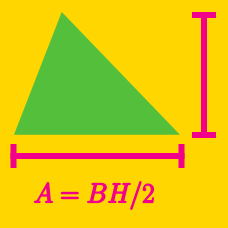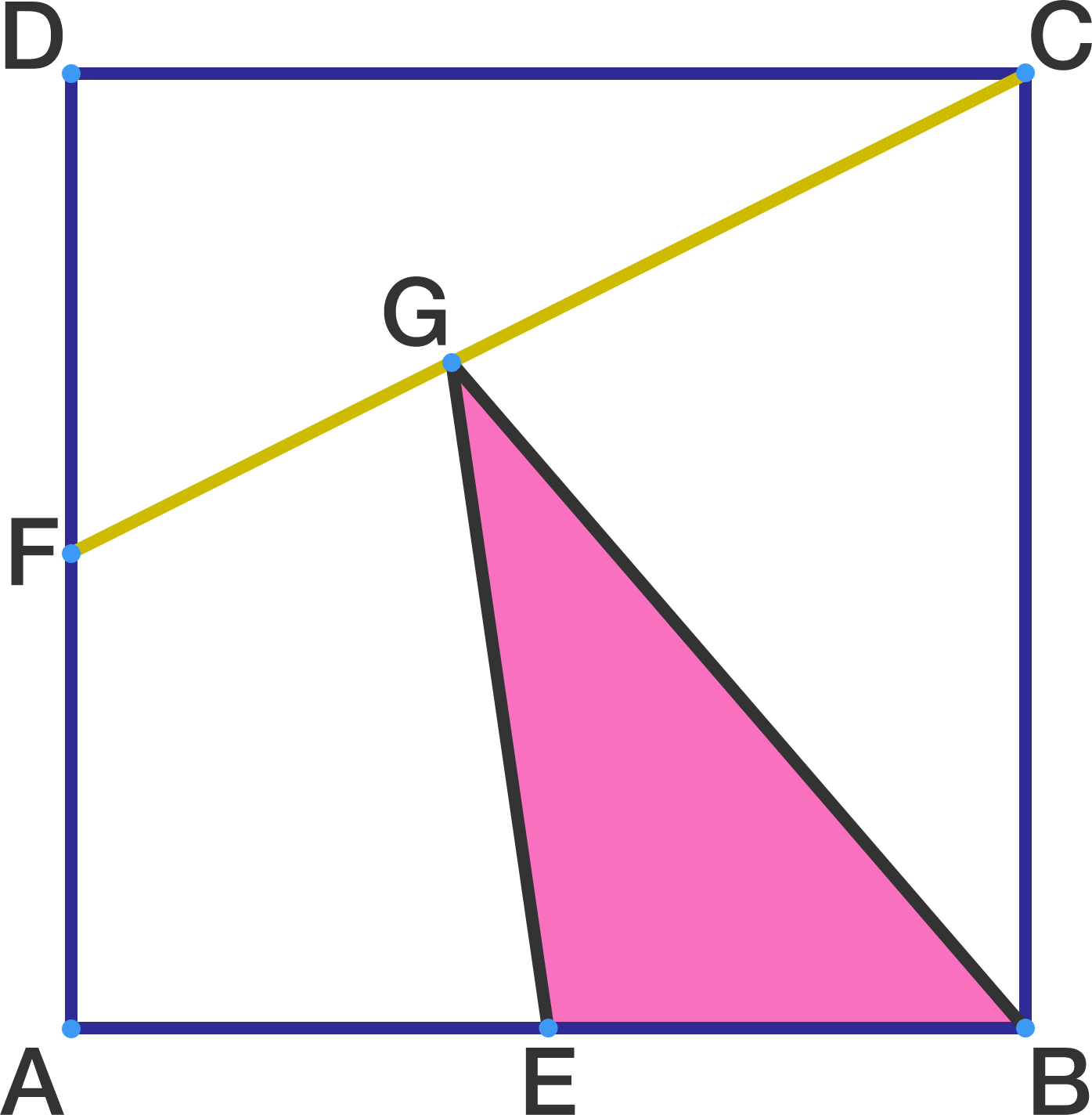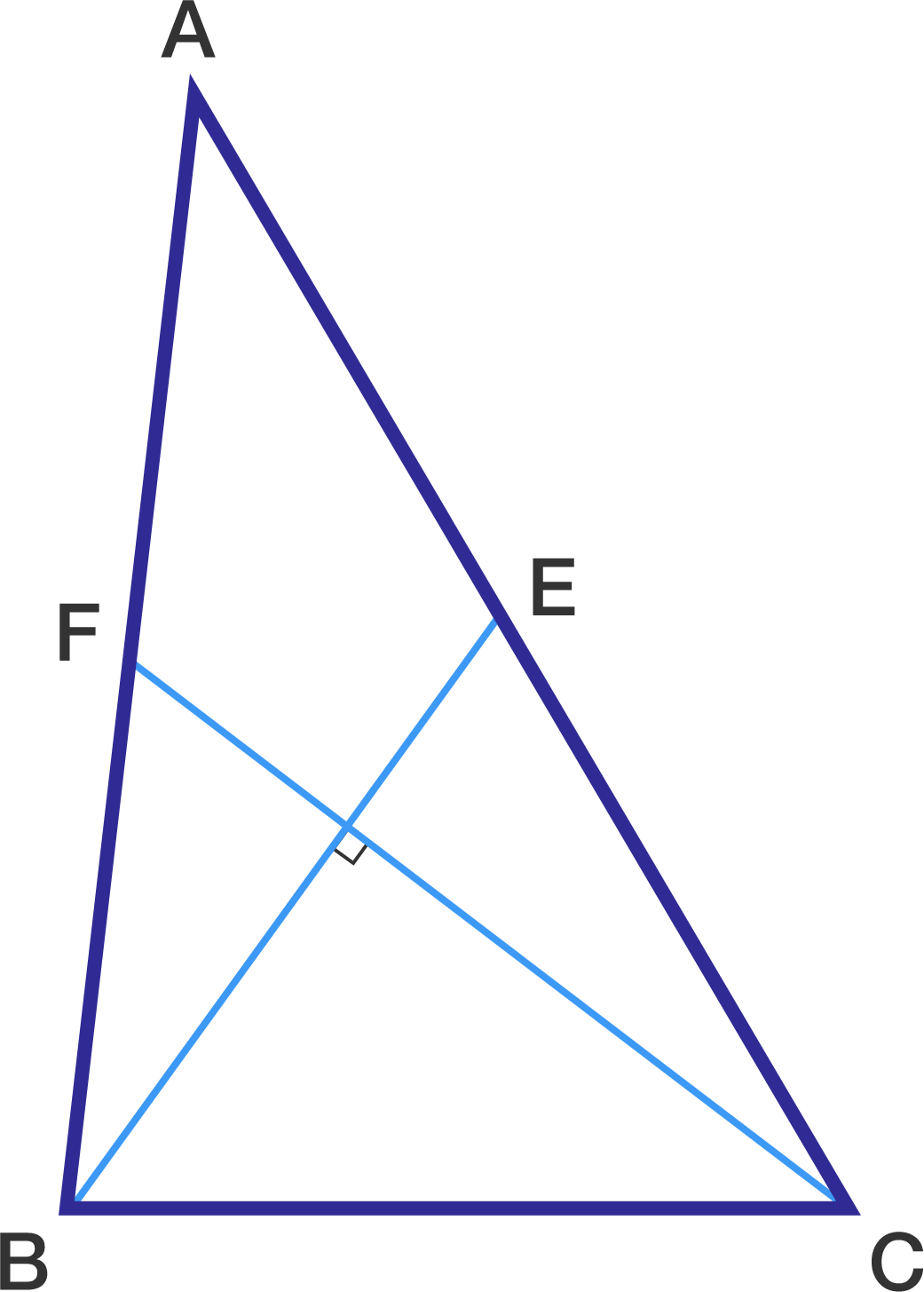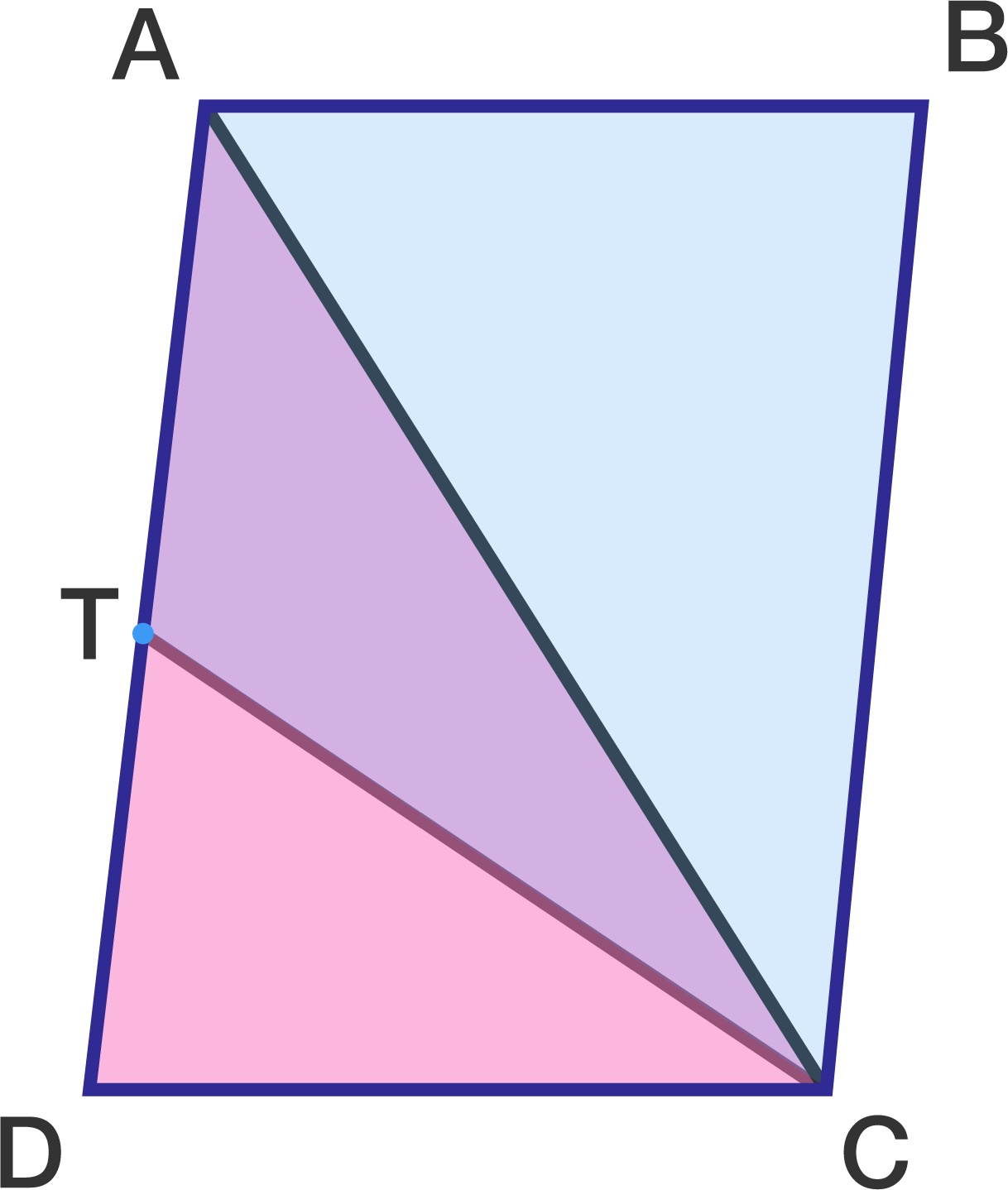Geometry

# Area of Triangles: Level 2 Challenges

Which of the following triangles has a larger area:

• triangle A with side lengths $13, 13, 10$, or
• triangle B with side lengths $13, 13, 24\, ?$In a square $ABCD$, $E$ and $F$ are the midpoints of sides $AB$ and $AD$, respectively. A point $G$ is taken on segment $CF$ in such a way that $2CG=3FG$.

If the side of the square is $2$, then what is the area of $\triangle{BEG}$?

Find the area of a triangle with sides $\sqrt{13}, \sqrt{61} , \sqrt{80}$.In triangle $\triangle ABC$, $BE$ and $CF$ are medians. $BE=9\text{ cm}$, $CF=12\text{ cm}$. If $BE$ is perpendicular to $CF$, find the area of the triangle $\triangle ABC$ in $\text{ cm}^2$.$ABCD$ is a parallelogram. The area of $ABCT$ is 45 and $T$ is the midpoint of $AD$. Find the area of triangle $ACD$.

×

Problem Loading...

Note Loading...

Set Loading...